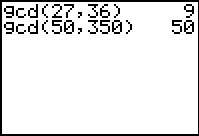# Knowledge Base

## Solution 18689: Calculating the gcd() (Greatest Common Divisor) on the TI-73 Family Graphing Calculators.

### How do I calculate the gcd() (Greatest Common Divisor) on the TI-73 family graphing calculators?

The greatest common divisor (GCD) function returns the largest number that divides into two positive whole numbers or lists of positive whole numbers evenly. If both arguments are lists, they must have the same number of elements. If one argument is a list and the other a non-list, the non-list is paired with each element of the list, and a list is returned.

Note: This is function is frequently used with fractions to reduce them to lowest terms on the TI-73 family.

The syntax is as follows:

gcd(valueA,valueB)

Examples:

• Find the greatest common divisor for the fraction, 27/36.

• Find the greatest common divisor for 50 and 350.

The gcd() function can be located by following the keystrokes provided below:

• Press [MATH]  for gcd(

• Input the values separated by a [,] and press [)] [ENTER]

Please see the examples provided below: